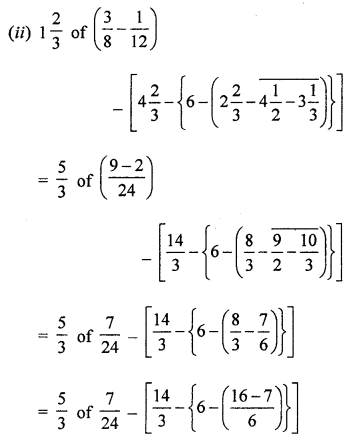## ML Aggarwal Class 7 Solutions for ICSE Maths Chapter 2 Fractions and Decimals Check Your Progress

Question 1.
What fraction is 270 gram of 3 kilograms?
Solution:Question 2.
Simplify the following:Solution:Question 3.
A shirt was marked at ₹ 540. It was sold at $$\frac { 3 }{ 4 }$$ of the marked price. What was the sale price?
Solution:
List price of the shirt = ₹ 540
Selling price = $$\frac { 3 }{ 4 }$$ of 540 = $$\frac { 3 }{ 4 }$$ × 540 = ₹ 405

Question 4.
In a class of 56 students, $$\frac { 1 }{ 4 }$$ are in blue house and $$\frac { 3 }{ 14 }$$ are in yellow house. Out of the remaining, $$\frac { 1 }{ 3 }$$ are in the greenhouse and the rest are in the red house. Find the number of students in each house.
Solution:
Number of total students of a class = 56
Number of students of blue house = $$\frac { 1 }{ 4 }$$ of 56 = 14
Number of students of yellow house = $$\frac { 3 }{ 14 }$$ of 56 = 12
Remaining students = 56 – (14 + 12) = 56 – 26 = 30
Number of students of green house = $$\frac { 1 }{ 3 }$$ of 30 = 10
and remaining students who are of red house = 30 – 10 = 20

Question 5.
Rohit bought a motorcycle for ₹ 36000. He paid $$\frac { 1 }{ 6 }$$ of the price in cash and the rest in 12 equal monthly instalments. Find the amount he had to pay every month.
Solution:
Cost price of motor cycle = ₹ 36000
Cash paid = $$\frac { 1 }{ 6 }$$ of ₹ 36000 = ₹ 6000
Remaining amount = ₹ 36000 – ₹ 6000 = ₹ 30000
Number of installments = 12
Amount of each installment = ₹ 30000 ÷ 12 = ₹ 2500
Rohit had to pay ₹ 2500 every month.

Question 6.
Mr Mukerjee gave $$\frac { 5 }{ 14 }$$ of his money to his son, $$\frac { 2 }{ 3 }$$ of the remainder to his daughter and the rest to his wife. If his wife got ₹ 36000, what was that total amount?
Solution:
Let total money = 1Question 7.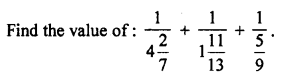Solution: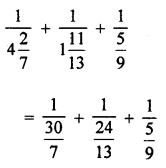Question 8.
Convert the following numbers to fractions (in simplest form):
(i) 0.025
(ii) 0.876
(iii) 4.3125
Solution: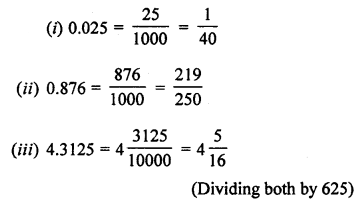Question 9.
Write the following fractions as decimals:
(i) 1$$\frac { 3 }{ 8 }$$
(ii) $$\frac { 47 }{ 125 }$$
(iii) 2$$\frac { 9 }{ 40 }$$
Solution: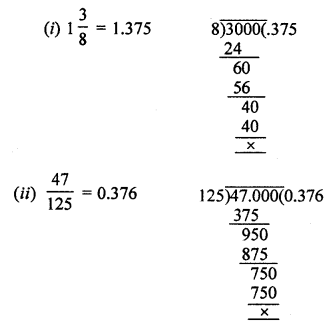Question 10.
By how much does the sum of 17.443 and 29.657 exceed the sum of 13.687 and 18.548?
Solution:
Sum of 17.443 and 29.567 = 17.443 + 29.657 = 47.100
and sum of 13.687 and 18.548 = 13.687 + 18.548 = 32.235
Difference = 47.100 – 32.235 = 14.865Question 11.
Simplify the following:
(i) 4.27 × 0.036
(ii) 0.09 × 1.04
(iii) 1.32 ÷ 0.8
(iv) 0.7038 ÷ 0.34
Solution:
(i) 4.27 × 0.036 = 0.15372(ii) 0.09 × 1.04 = 0.0936
(iii) 1.32 ÷ 0.8 ⇒ 1.32 ÷ 0.80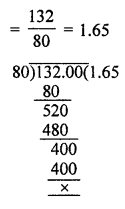(iv) 0.7038 ÷ 0.34 ⇒ 0.7032 ÷ 0.3400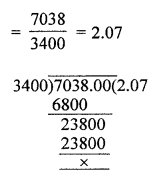Question 12.
If one kg of rice costs ₹ 52.70, then find the cost of 12.5 kg rice.
Solution:
Cost of 1 kg of rice = ₹ 52.70
Then cost of 12.5 kg of rice = ₹ 52.70 × 12.5 = ₹ 658.75 = ₹ 658.75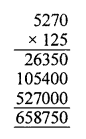Question 13.
A piece of cloth is 24.5 m long. How many pieces, each of length 1.75 m, can be cut from it?
Solution:
Length of a piece of cloth = 24.5 m
Length of one piece of cloth cut from it = 1.75 m
Number of piece = 24.5 ÷ 1.75 = 24.50 ÷ 1.75 = 14 piecesQuestion 14.
The product of two decimal numbers is 1.599 and one of them ¡s 0.65, find the other.
Solution:
Product of two decimal number = 1.599
One Number = 0.65Question 15.
Simplify the following:Solution: